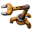## Return variance of benchmark runs

Post your ideas and suggestions how to improve the game.
Allaizn
InserterPosts: 41
Joined: Sat Mar 03, 2018 12:07 pm
Contact:

### Return variance of benchmark runs

The benchmark command line option is extremely useful when trying to compare the relative performance of build, but it has a major downfall:
Currently, only the average, minimal and maximal update times are reported.
Both the minimal and maximal update times are pretty much useless, since any one anomalous tick distorts this value.
It's much more useful to report the Variance, or it's square root named the Standard Deviation
##### Why is this useful?
Doing a benchmark run over say 10'000 ticks results in a measurement of say 123'456.789 ms. This may lead one to think that 1 tick takes exactly 123.456789ms on average, since that's the accuracy that's reported.
But repeating the benchmark multiple times shows that that's not the case: different runs will vary the timing. Let's say 4 further runs were made and the following results were obtained: 123'486.523ms, 123'389.889ms, 123'534.358ms and 123444.048ms.
These show that the measured average is not that precise, only the first three digits seem be be correct, but how can we calculate this fact?
The answer lies in statistical mathematics and is called the variance, which measures how far a set of numbers spreads around its average. It's calculated by averaging the squares of the differences between the values and their shared mean. Due to mathematical technicalities, we don't divide the total sum by 5 (since that's the number of measurements), but instead need to divide by one less, namely 4. We can also ask wolframalpha to do that calculation for us, which turns out to be 2846.49ms². The square in the units is of course bothersome, which motivates the definition of the standard deviation simply being the squre root of that, or 53.352ms
To summarize: the average of the 5 measurements is 123'462.321ms with a standard deviation of 53.352ms.
These values can then be used to calculate the Margin of Error. For example using a 95% confidence interval results in the above experiment to conclude with a result of 123'462.321±46.764ms for 10'000 ticks, or 12.346±0.005ms for a single one.
That result is now much more meaningful, since it tells us exactly how precise the measurement was!
But achieving this result currently requires one to run the benchmark multiple times. It's much more meaningful to run 5 benchmarks for 10'000 ticks than to run a single 50'000 tick one, which is of course ridiculous! This is all because the variance is not gathered/ reported, which is thankfully not that much more work to do:
##### How to calculate the variance on the fly
There are multiple algorithms that can be used to calculate the variance, but most require the knowledge of the average (which we of course don't have while the benchmark is running!).
Thankfully, there's a numerically stable online algorithm, that allows us to calculate the variance without the need of storing all individual timings. Some C++-ish code (it's been a while since I wrote something in that language):

Code: Select all

``````class Timer {
int runningTotal = 0;
double runningVariance = 0;
int ticksSoFar = 0;

void updateWithNextUpdateDuration(int durationInMuS) {
double oldMean = ticksSoFar > 0 ? runningTotal / ticksSoFar : runningTotal;
runningTotal += durationInMuS;
ticksSoFar += 1;
double newMean = runningTotal / tickSoFar;
runningVariance += (durationInMuS - oldMean) * (durationInMuS - newMean)
}

string reportResult() {
return "total time: " + (runningTotal / 1000.0).to_string("0.000") + "ms, "
+ "avg: " + (runningTotal / (1000.0 * ticksSoFar)).to_string("0.000") + "ms, "
+ "stanDev: " + (sqrt(runningVariance / (ticksSoFar - 1)) / 1000.0).to_string("0.000") + "ms"
}
}
``````
Finally, I'd like to mention my benchmarking tutorial, where you'll be able to see another example of the calculation that's currently necessary to work around the missing of this small but incredibly useful feature.

### Who is online

Users browsing this forum: No registered users and 3 guests